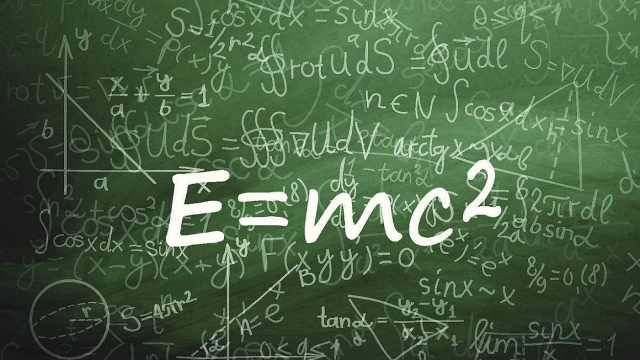$$e=mc^2$$

# Theorem

The energy imparted to a body to cause that body to move causes the body to increase in mass by a value 𝑀 as given by the equation:
$$E = M c^2$$
where 𝑐 is the speed of light.

# Proof

From Einstein’s Law of Motion, we have:
$$\mathbf F = \dfrac {m_0 \mathbf a} {\left({1 - \dfrac {v^2} {c^2}}\right)^{\tfrac 3 2}}$$
where:

• 𝐅 is the force on the body
• 𝐚 is the acceleration induced on the body
• 𝑣 is the magnitude of the velocity of the body
• 𝑐 is the speed of light
• 𝑚0 is the rest mass of the body.
Without loss of generality, assume that the body is starting from rest at the origin of a cartesian coordinate plane.

Assume the force 𝐅 on the body is in the positive direction along the x-axis.

To simplify the work, we consider the acceleration as a scalar quantity and write it 𝑎.

Thus, from the Chain Rule:
$$a = \dfrac{\mathrm d v}{\mathrm d t} = \dfrac{\mathrm d v}{\mathrm d x} \dfrac {\mathrm d x}{\mathrm d t} = v \dfrac {\mathrm d v} {\mathrm d x}$$

Then from the definition of energy:
$$\displaystyle E = \int_0^x F \mathrm d x$$
$$E = m_0 \int_0^x \frac a {\left({1 - v^2 / c^2}\right)^{\tfrac 3 2} } \ \mathrm d x$$
$$= m_0 \int_0^v \frac v {\left({1 - v^2 / c^2}\right)^{\tfrac 3 2} } \ \mathrm d v$$
$$= m_0 \left({- \frac {c^2} 2}\right) \int_0^v \left({1 - \frac {v^2} {c^2} }\right)^{-\tfrac 3 2} \left({- \frac {2 v \ \mathrm d v} {c^2} }\right)$$
$$= \left[{m_0 c^2 \left({1 - \frac {v^2} {c^2} }\right)^{- \tfrac 1 2} }\right]_0^v$$
$$= m_0 c^2 \left({\frac 1 {\sqrt {1 - \frac {v^2} {c^2} } } - 1}\right)$$
$$= c^2 \left({\frac {m_0} {\sqrt {1 - \frac {v^2} {c^2} } } - m_0}\right)$$
$$= c^2 \left({m - m_0}\right)$$
$$= M c^2$$